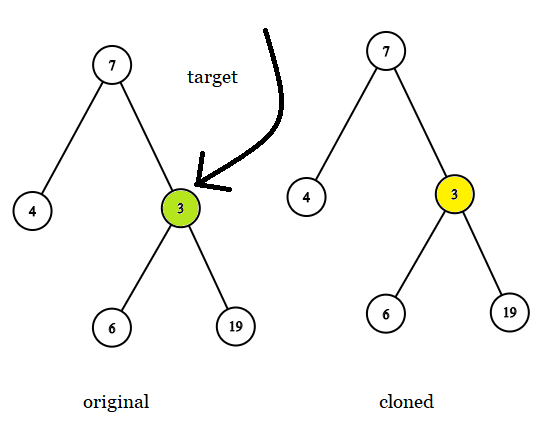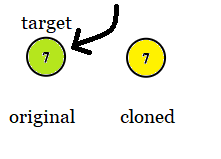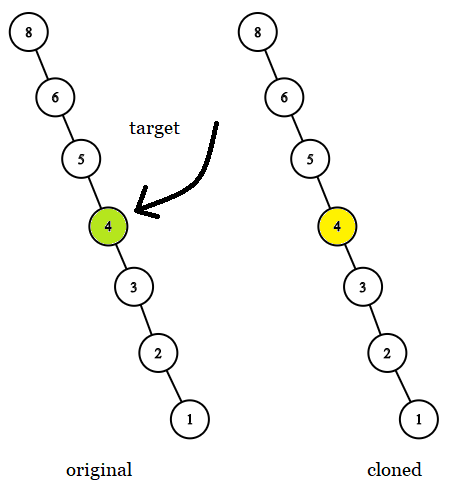# [Leetcode]1379. Find a Corresponding Node of a Binary Tree in a Clone of That Tree

limelimejiwon·2022년 5월 18일
0

## Python으로 공부하는 코딩테스트

목록 보기
53/67## 📄 Description

Given two binary trees original and cloned and given a reference to a node target in the original tree.

The cloned tree is a copy of the original tree.

Return a reference to the same node in the cloned tree.

Note that you are not allowed to change any of the two trees or the target node and the answer must be a reference to a node in the cloned tree.

### Example 1:Input: tree = [7,4,3,null,null,6,19], target = 3
Output: 3
Explanation: In all examples the original and cloned trees are shown. The target node is a green node from the original tree. The answer is the yellow node from the cloned tree.

### Example 2:Input: tree = , target =  7
Output: 7

### Example 3:Input: tree = [8,null,6,null,5,null,4,null,3,null,2,null,1], target = 4
Output: 4

### Constraints:

• The number of nodes in the tree is in the range $[1, {10^4}]$.
• The values of the nodes of the tree are unique.
• target node is a node from the original tree and is not null.

## 💻 My Submission - using Stack

class Solution:
def getTargetCopy(self, original: TreeNode, cloned: TreeNode, target: TreeNode) -> TreeNode:
c_start=cloned
stack=[c_start]
while stack:
node=stack.pop()
if node.val==target.val:
return node
if node.left:stack.append(node.left)
if node.right:stack.append(node.right)

## 🎈 DFS - using Recursion

def getTargetCopy(self, original: TreeNode, cloned: TreeNode, target: TreeNode) -> TreeNode:
def find_node(orignal,copy,find):
if not orignal:
return None
if orignal == find:
return copy
return find_node(orignal.left,copy.left, find) or find_node(orignal.right,copy.right,find)
return find_node(original,cloned,target)`

References